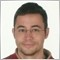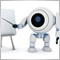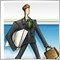From SMA or EMA to f(x)3577

Hi there!,

Is there an existing way to convert the signal of a simple or exponential moving average to a math function of one variable?

Thank you very much in advance39055

laplacianlab:

Hi there!,

Is there an existing way to convert the signal of a simple or exponential moving average to a math function of one variable?

Thank you very much in advance

Which variable ? Why do that ?3577

angevoyageur:
Which variable ? Why do that ?
That would be very versatile. Many things could be simplified, I think, for example, the power of a trend would be the slope of the function at a given point.39055

laplacianlab:
That would be very versatile. Many things could be simplified, I think, for example, the power of a trend would be the slope of the function at a given point.
Interesting dream ;-) But if anyone have this, I don't believe they share here.Moderator
1956

angevoyageur:
Interesting dream ;-) But if anyone have this, I don't believe they share here.

Or we can fulfill this idea in an alternative way.

Computing the tag() of two bars' MA,

eg:

tag(A) = (SMA(1)-SMA(2))/ B242

I know indicator sma different than ema. But i do not understand meaning sma,ema and application of them.4750

hoan:
I know indicator sma different than ema. But i do not understand meaning sma,ema and application of them.

it return to the weight of the new and old data for calculate " average".

in ema the new data or " bar" has bigger weight .3577

luenbo:

Or we can fulfill this idea in an alternative way.

Computing the tag() of two bars' MA,

eg:

tag(A) = (SMA(1)-SMA(2))/ B

Thanks for your reply, now I see this is a bit more complicated that I originally thought, but still interesting anyway.242

thanks for clearly explain . i understand but i do not know use sma ema in my chart to trade39055

hoan:
thanks for clearly explain . i understand but i do not know use sma ema in my chart to trade
You have to learn from here
School of Pipsology Moving Averages
• www.babypips.com
Thinking of trading in a trending environment? Try using moving averages! Lessons on Moving Averages Silky Smooth Moving Averages A moving average is simply a way to smooth out price action over time. Here's what it looks like. Simple Moving Averages These are calculated by adding up the last "X" period's closing prices and...3577

angevoyageur:
Interesting dream ;-) But if anyone have this, I don't believe they share here.

Hello there!,

Well, in fact I've discovered that it is not a dream! You can obtain a math function of one variable through Lagrange Interpolating Polynomials, for instance, cause there are several ways, but this is a hard work that should be explained in an article, I think.

For those of you interested in this problem, please, have a look at this

http://mathworld.wolfram.com/LagrangeInterpolatingPolynomial.html

Lagrange Interpolating Polynomial -- from Wolfram MathWorld
• mathworld.wolfram.com
The Lagrange interpolating polynomial is the polynomial of degree that passes through the points , , ..., , and is given by The formula was first published by Waring (1779), rediscovered by Euler in 1783, and published by Lagrange in 1795 (Jeffreys and Jeffreys 1988). When constructing interpolating polynomials, there is a tradeoff...